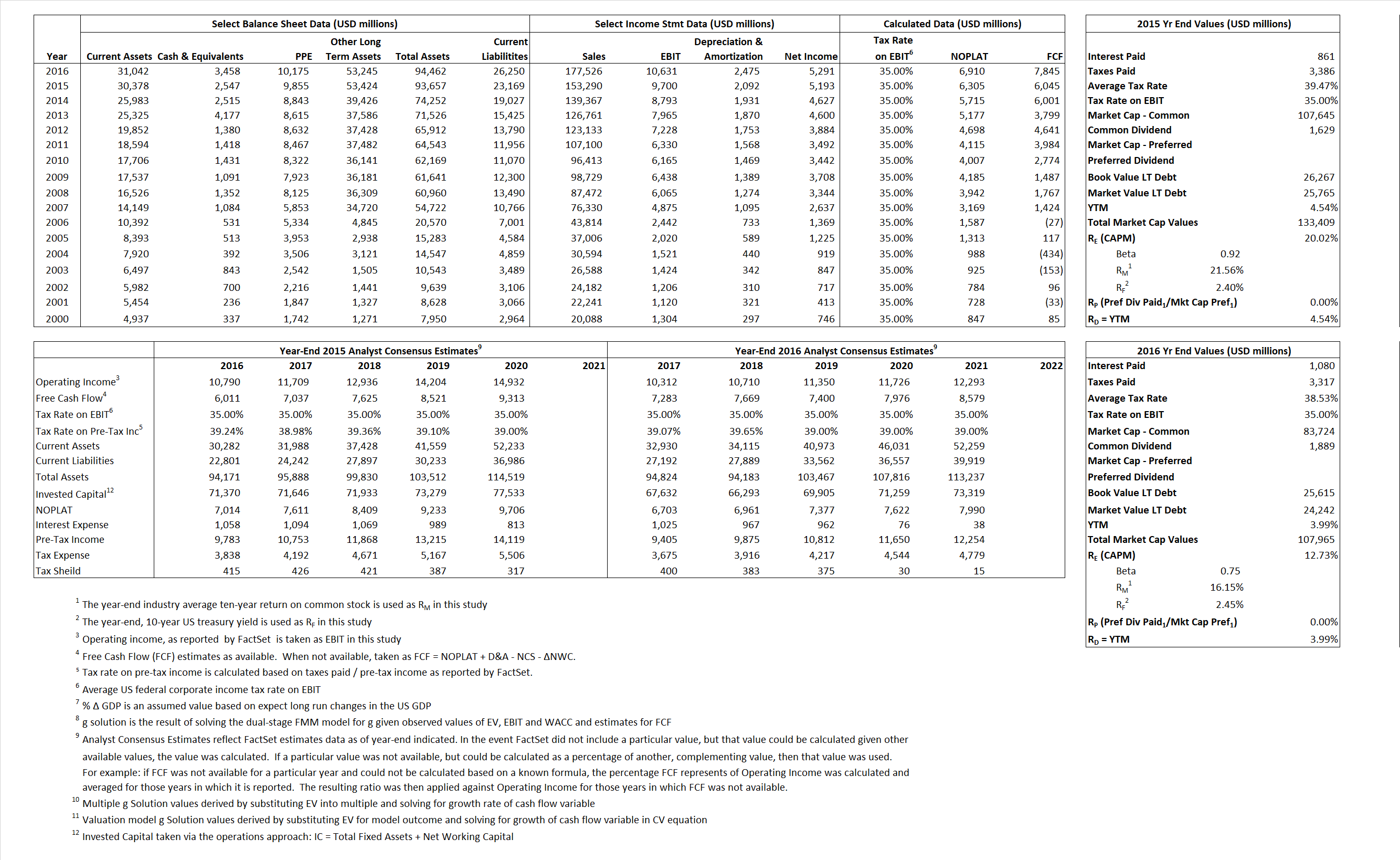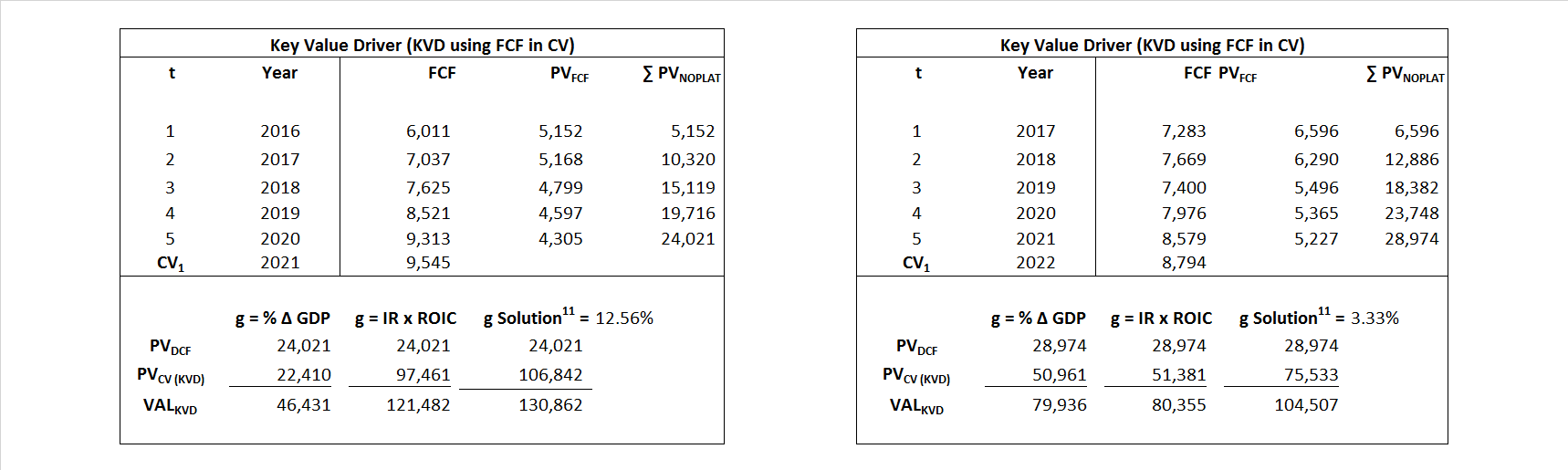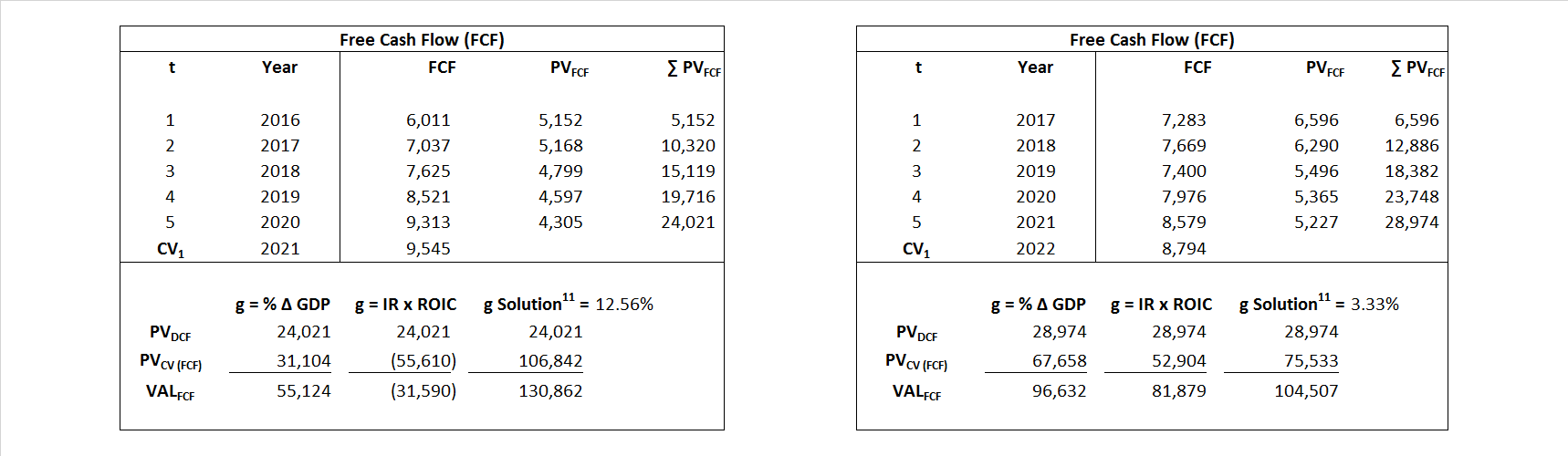# CVS

## Analyst Listing

The following analysts provide coverage for the subject firm as of May 2016:

 Broker Analyst Analyst Email Stephens Inc Ben Wyatt ben.wyatt@stephens.com Susquehanna Financial Group Biju Perincheril biju.perincheril@sig.com Johnson Rice & Company Charles Meade cmeade@jrco.com Cowen & Company Charles Robertson charles.robertson@cowen.com Piper Jaffray David Kistler david.w.kistler@simmonspjc.com Wells Fargo Securities David Tameron david.tameron@wellsfargo.com GMP Securities Derrick Whitfield dwhitfield@gmpsecurities.com Credit Suisse Edward Westlake edward.westlake@credit-suisse.com IBERIA Capital Partners Eric Fox eric.fox@iberiabank.com Alembic Global Advisors James Sullivan james.sullivan@alembicglobal.com KLR Group John Gerdes jjg@klrgroup.com Jefferies Jonathan D. Wolff jwolff@jefferies.com Raymond James Kevin Smith kevin.smith@raymondjames.com Nomura Research Lloyd Byrne lloyd.byrne@nomura.com Jefferies Mark Wiltamuth mwiltamuth@jefferies.com Seaport Global Securities Michael Kelly mkelly@seaportglobal.com Mitsubishi UFJ Securities (USA) Michael McAllister mmcallister@us.sc.mufg.jp Oppenheimer Mohan Naidu mohan.naidu@opco.com Wolfe Research Paul Sankey psankey@wolferesearch.com Scotia Howard Weil Peter Kissel peter.kissel@scotiabank.com BMO Capital Markets Phillip Jungwirth phillip.jungwirth@bmo.com Capital One Securities Phillips Johnston phillips.johnston@capitalone.com Deutsche Bank Research Ryan Todd ryan.todd@db.com RBC Capital Markets Scott Hanold scott.hanold@rbccm.com Evercore ISI Stephen Richardson stephen.richardson@evercoreisi.com Guggenheim Securities Subash Chandra subash.chandra@guggenheimpartners.com Wunderlich Securities Vedran Vuk vedranvuk@wundernet.com Mizuho Securities USA Ann Hynes ann.hynes@us.mizuho-sc.com Cowen & Company Charles Rhyee charles.rhyee@cowen.com RBC Capital Markets David Francis david.francis@rbccm.com Leerink Partners David Larsen david.larsen@leerink.com SunTrust Robinson Humphrey David S. MacDonald david.macdonald@suntrust.com Deutsche Bank Research George Hill george.hill@db.com Guggenheim Securities John Heinbockel john.heinbockel@guggenheimpartners.com Raymond James John W. Ransom john.ransom@raymondjames.com Jefferies Mark Wiltamuth mwiltamuth@jefferies.com Oppenheimer Mohan Naidu mohan.naidu@opco.com Wells Fargo Securities Peter Costa peter.costa@wellsfargo.com Credit Suisse Robert M. Willoughby robert.willoughby@credit-suisse.com Atlantic Equities Sam Hudson s.hudson@atlantic-equities.com Wolfe Research Scott Mushkin smushkin@wolferesearch.com FBR Capital Markets & Co Steven Halper shalper@fbr.com

## Primary Input Data## Derived Input Data

### Equational Form

Net Operating Profit Less Adjusted Taxes NOPLAT    6,305 6,910$NOPLAT\, =\, EBIT\, x\, (1 \,-\, Avg \,\,Tax\,\, Rate\,\, on\,\, EBIT)$
Free Cash Flow FCF  6,045 7,845$FCF\,=NOPLAT\,+\,Non-Cash\,Expenses-\Delta NWC\,-\,NCS$
Tax Shield TS  340  416$TS\,=\,Interest\,\,Paid\,\,x\,\, Avg \,\,Tax\,\,Rate\,\, on\,\, Pre-Tax\,\, Income$
Invested Capital IC  70,488  68,212$IC\,=\,Fixed\,\,Operating\,\,Assets\,\,+\,\,Net\,\, Working\,\, Capital$
Return on Invested Capital ROIC 70,488 10.13%$ROIC\,=\,\frac { NOPLAT }{ IC }$
Net Investment NetInv  17,355 199$NetInv\,=\,{ {IC}_{1}}-{{IC}_{0}}+Depreciation$
Investment Rate IR 275.26% 2.88%$IR\,=\,\frac {NetInv}{NOPLAT}$
Weighted Average Cost of Capital WACCMarket 16.69% 10.42%$WACC\,=\,\frac { E }{ V } { R }_{ E }\,+\,\frac { P }{ V } { R }_{ P }\,+\,\frac { D }{ V } { R }_{ D }\left( 1- Avg\,\, Tax\,\,Rate\,\,on\,\,Pre-Tax\,\,Income \right)$
WACCBook 7.39% 9.44%
Enterprise value EVMarket 130,862 104,507$EV\,=\,Market\,\,Cap\,\,Equity\,+\,\,Long\,\,Term\,\,Debt\,-\,Cash$
EVBook 131,365  105,881
EV/EBIT Multiple$\frac{EV_{Market}}{EBIT}$ 13.49  9.83$EV/EBIT\,=\,\frac { EV}{ EBIT}$
Long-Run Growth g = IR x ROIC
24.62%   0.29% Long-run growth rates of the income variable  are used in the Continuing Value portion of the valuation models.
g = %$\Delta$ GDP   2.50%   2.50%

## Valuation Model Outcomes

The outcomes presented in this study are the result of original input data, derived data, and synthesized inputs and, depending on the equational form of any particular valuation model, may result in irrelevant or implausible results.  For example, in the event WACC < g, the value of this term, often found in the denominator of an equation’s continuation value term, will be expressly negative and may result in a negative overall valuation for the firm.  In the event of a WACC < g relation, the model form as applied to the subject firm offers an irrelevant outcome.

### Equational form

Key Value Driver (NOPLAT) KVD (NOPLAT)${ Value }_{ DCF/KVD }=\sum { \frac { NOPLAT_{ t } }{ { \left( 1+WACC \right) }^{ t } } +\frac { \frac { { NOPLAT }_{ 1 }\left( 1-\frac { g }{ ROIC } \right) }{ WACC-g } }{ { \left( 1+WACC \right) }^{ t } } }$Key Value Driver (FCF) KVD (FCF)${ Value }_{ DCF/KVD }=\sum { \frac { FCF_{ t } }{ { \left( 1+WACC \right) }^{ t } } +\frac { \frac { { NOPLAT }_{ 1 }\left( 1-\frac { g }{ ROIC } \right) }{ WACC-g } }{ { \left( 1+WACC \right) }^{ t } } }$Free Cash Flow FCF${ Value }_{ DCF/FCF }=\sum { \frac { FCF_{ t } }{ { \left( 1+WACC \right) }^{ t } } +\frac { \frac { { FCF }_{ 1 }}{ WACC-g } }{ { \left( 1+WACC \right) }^{ t } } }$Economic Profit ECON π${ Value }_{ { ECON\pi } }= I{ C }_{ 0 }+\sum { \frac { { IC }_{ t-1 }(ROI{ C }_{t}-WAC{C}_{t}) }{ { \left( 1+WACC \right) }^{ t } }+ \frac {\frac { I{C}_{0}\ x\ (ROI{C}_{1}\ -\ WAC{C}_{1}) }{ WACC-g } }{ { \left( 1+WACC \right) }^{ t } } }$Adjusted Present Value APV${ Value }_{ APV }=\sum { \frac { FCF_{ t } }{ { \left( 1+{ k }_{ u } \right) }^{ t } } +\frac { \frac { { FCF }_{ 1 }}{ { k }_{ u }-g } }{ { \left( 1+{ k }_{ u } \right) }^{ t } } } +\sum { \frac { { TS }_{ t } }{ { \left( 1+{ k }_{ tax } \right) }^{ t } } +\frac { \frac { { TS }_{ 1 }}{ { k }_{ tax }-g } }{ { \left( 1+{ k }_{ tax } \right) }^{ t } } }$Forward Market Multiple FMM${ Value }_{ DCF/FMM}=\sum { \frac { FCF_{ t } }{ { \left( 1+WACC \right) }^{ t } } +\frac { { EBIT }_{ 1 }\,{x}\,{FMM}}{ { \left( 1+WACC \right) }^{ t } } }{\,\,\,; \,\,FMM\,=\,\frac{{EV}_{t=0}}{{EBIT}_{t=0}}}$